# Nevanlinna-Pick interpolation

Jump to: navigation, search

Nevanlinna–Pick interpolation problem

The data for the classical Nevanlinna–Pick interpolation problem [a13], [a15] consist ofdistinct points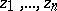in the open unit disc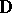and complex numbers. Given these data, the problem is to find (if possible) a function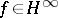, the Hardy space of all bounded analytic functions on(cf. also Hardy classes), such that:

a)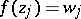for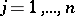;

b). Instead of condition b) one may require the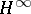norm of the interpolantto be strictly less than one, and in the latter case one calls the problem suboptimal. The problem may also be considered for infinite collections of points ([a14]).

The Nevanlinna–Pick interpolation problem is solvable if and only if the associated Pick matrix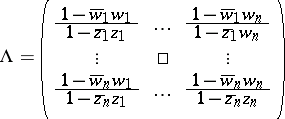is positive semi-definite, and its solution is unique if and only if, in addition,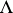is singular. For the suboptimal version of the problem to be solvable it is necessary and sufficient that the Pick matrixbe positive definite, and in this case there is a rational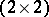-matrix function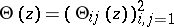such that the infinitely many solutionsof the problem are described bywhere the free parameteris an arbitrary-function with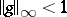. Moreover, forone may take (see [a2], Sect. 18.1) the matrix function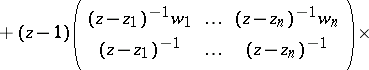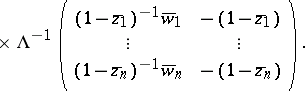Matrix- and operator-valued versions of the Nevanlinna–Pick problem, including one-sided and two-sided tangential variants, have also been introduced, and many different approaches have been developed to treat the problem and its various generalizations. For the history of the subject, see [a2], [a7], [a8], [a16], [a12].

The Nevanlinna–Pick interpolation theorems and their matrix generalizations have proved to be very useful in solving engineering problems, in particular, in circuit theory [a4] and for control theory problems involving an-criterion (see [a10] and [a6], Chapt. 9). This development led to a new field of research, in which the emphasis is on interpolants that are rational matrix functions and on the search for explicit formulas and efficient algorithms for the construction of interpolants in a form which is suitable for engineering applications (see [a2], Part VI, and [a18]).

The Nevanlinna–Pick problem also has a non-stationary version, in which the role of analytic functions is taken over by lower-triangular operators and points in the open unit disc are replaced by weighted shifts with spectral radius strictly less than one. To give an example, let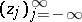and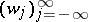be two bounded sequences of complex numbers, and assume that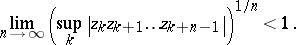The non-stationary Nevanlinna–Pick interpolation problem associated with these data is to find a lower-triangular operator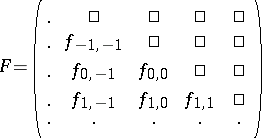with operator norm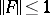such that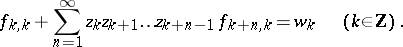Several methods to treat such non-stationary interpolation problems have been developed (see [a11], [a5] and [a17]); the main results for the stationary case carry over to the non-stationary case (cf. [a9]).

Nevanlinna–Pick interpolation problems have also been solved for analytic functions on a half-plane. A non-stationary analogue of the latter may be found in [a1]. Generalizations of the Nevanlinna–Pick interpolation theory have also been developed for functions of several variables (see [a16], notes to Chapt. 2) and in a non-linear setting [a3].